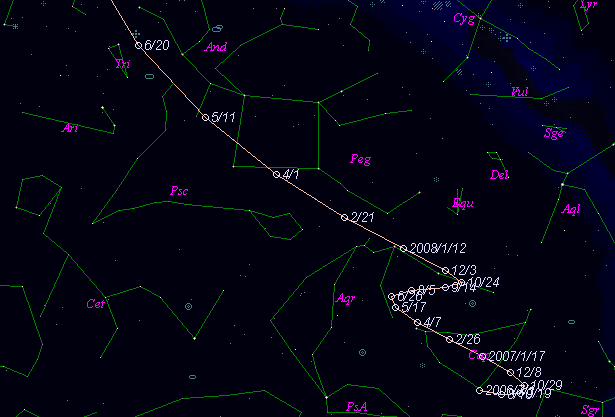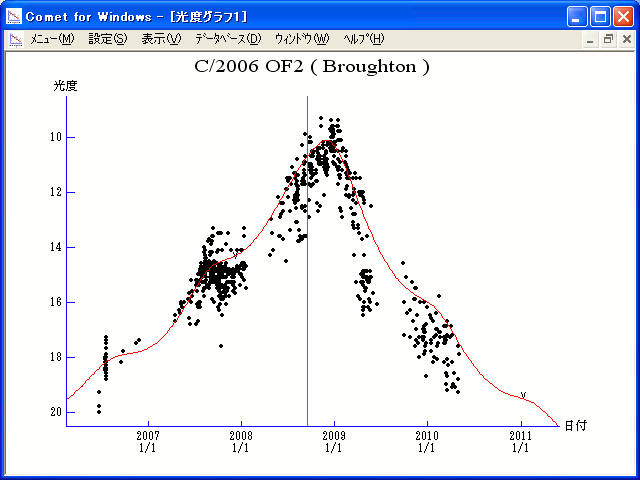# \$B%V%m!<%H%sWB@1(B

C/2006 OF2 ( Broughton )###\$B%W%m%U%#!<%k(B

 \$BH/8+F|(B 2006\$BG/(B7\$B7n(B17\$BF|(B \$BH/8+8wEY(B 18.2\$BEy(B \$BH/8+ J. Broughton (Reedy Creek)

###\$B###\$B50F;MWAG(B

```Epoch 2008 Sept. 11.0 TT = JDT 2454720.5
T 2008 Sept. 15.68492 TT                                MPC
q   2.4314426            (2000.0)            P               Q
z  -0.0003518      Peri.   95.61245     +0.49676861     -0.80147061
+/-0.0000000      Node   318.50772     +0.45178297     +0.56638061
e   1.0008555      Incl.   30.17047     +0.74102165     +0.19198404
From 4534 observations 2006 June 23-2010 May 11, mean residual 0".6.
```

###\$B@1?^(B###\$B8wEYJQ2=(B

```        m1 = 5.0 + 5 log\$B&\$(B + 10.0 log r(t - 50)  [  ,50]  (              \$B!A(B2008\$BG/(B11\$B7n(B 4\$BF|(B)
m1 = 4.0 + 5 log\$B&\$(B + 12.5 log r(t - 50)  [50,  ]  (2008\$BG/(B11\$B7n(B 4\$BF|!A(B              )
```##### \$B50F;MWAG\$O(BM.P.E.C. 2013-B90\$B\$K7G:\\$5\$l\$?\$b\$N\$G\$9!#(B \$B@1?^\$O(B StellaNavigator Ver.2.0 for Windows (\$B%"%9%H%m%"!<%D(B \$BJTCx(B / \$B%"%9%-!<=PHG6I4)(B) \$B\$G:n@.\$7\$?\$b\$N\$G\$9!#(B \$B8wEY%0%i%U\$O(BComet for Windows\$B\$G:n@.\$7\$?\$b\$N\$G\$9!#(B